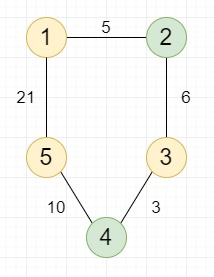# Find the shortest distance between any pair of two different good nodes in C++

Suppose we have a given weighted undirected graph with N different nodes and M edges, some of the nodes are good nodes. We have to find the shortest distance between any pair of two different good nodes. In the given diagram the yellow in the following graph are considered to be good nodes.

So, if the input is likethen the output will be 11, as the pairs of good nodes and distance between them are: (1 to 3) the distance is 11, (3 to 5) the distance is 13, (1 to 5) the distance is 24, out of which 11 is the minimum.

To solve this, we will follow these steps −

• N := 100005

• MAX_VAL := 99999999

• create one priority queue q

• result := MAX_VAL

• for initialize i := 1, when i <= n, update (increase i by 1), do −

• if good_verts[i] is false, then −

• for initialize j := 1, when j <= n, update (increase j by 1), do −

• dist[j] := MAX_VAL

• vis[j] := 0

• dist[i] := 0

• while (not q is empty), do −

• delete element from q

• insert { 0, i } into q

• good := 0

• while (not q is empty), do −

• v := top element of q

• delete element from q

• if vis[v] is true, then −

• vis[v] := 1

• good := good + (1 when good_verts[v] is true, otherwise 0)

• if dist[v] > result, then −

• Come out from the loop

• if good is same as 2 and good_verts[v], then −

• result := minimum of result and dist[v]

• Come out from the loop

• for initialize j := 0, when j < size of graph[v], update (increase j by 1), do −

• to := graph[v, j].first

• weight := graph[v, j].second

• if dist[v] + weight < dist[to], then −

• dist[to] := dist[v] + weight

• insert { dist[to], to } into q

• return result

## Example

Let us see the following implementation to get better understanding −

Live Demo

#include <bits/stdc++.h>
using namespace std;
#define N 100005
#define MAX_VAL 99999999
void insert_edge(vector<pair<int, int> > graph[], int x, int y, int weight) {
graph[x].push_back({ y, weight });
graph[y].push_back({ x, weight });
}
int get_min_dist(vector<pair<int, int> > graph[], int n, int dist[], int vis[], int good_verts[], int k) {
priority_queue<pair<int, int>, vector<pair<int, int> >, greater<pair<int, int>>> q;
int result = MAX_VAL;
for (int i = 1; i <= n; i++) {
if (!good_verts[i])
continue;
for (int j = 1; j <= n; j++) {
dist[j] = MAX_VAL;
vis[j] = 0;
}
dist[i] = 0;
while (!q.empty())
q.pop();
q.push({ 0, i });
int good = 0;
while (!q.empty()) {
int v = q.top().second;
q.pop();
if (vis[v])
continue;
vis[v] = 1;
good += good_verts[v];
if (dist[v] > result)
break;
if (good == 2 and good_verts[v]) {
result = min(result, dist[v]);
break;
}
for (int j = 0; j < graph[v].size(); j++) {
int to = graph[v][j].first;
int weight = graph[v][j].second;
if (dist[v] + weight < dist[to]) {
dist[to] = dist[v] + weight;
q.push({ dist[to], to });
}
}
}
}
return result;
}
int main() {
int n = 5, m = 5;
vector<pair<int, int> > graph[N];
insert_edge(graph, 1, 2, 3);
insert_edge(graph, 1, 2, 3);
insert_edge(graph, 2, 3, 4);
insert_edge(graph, 3, 4, 1);
insert_edge(graph, 4, 5, 8);
int k = 3;
int good_verts[N], vis[N], dist[N];
good_verts = good_verts = good_verts = 1;
cout << get_min_dist(graph, n, dist, vis, good_verts, k);
}

## Input

n = 5, m = 5
insert_edge(graph, 1, 2, 3);
insert_edge(graph, 1, 2, 3);
insert_edge(graph, 2, 3, 4);
insert_edge(graph, 3, 4, 1);
insert_edge(graph, 4, 5, 8);
k = 3
good_verts = good_verts = good_verts = 1;

## Output

7